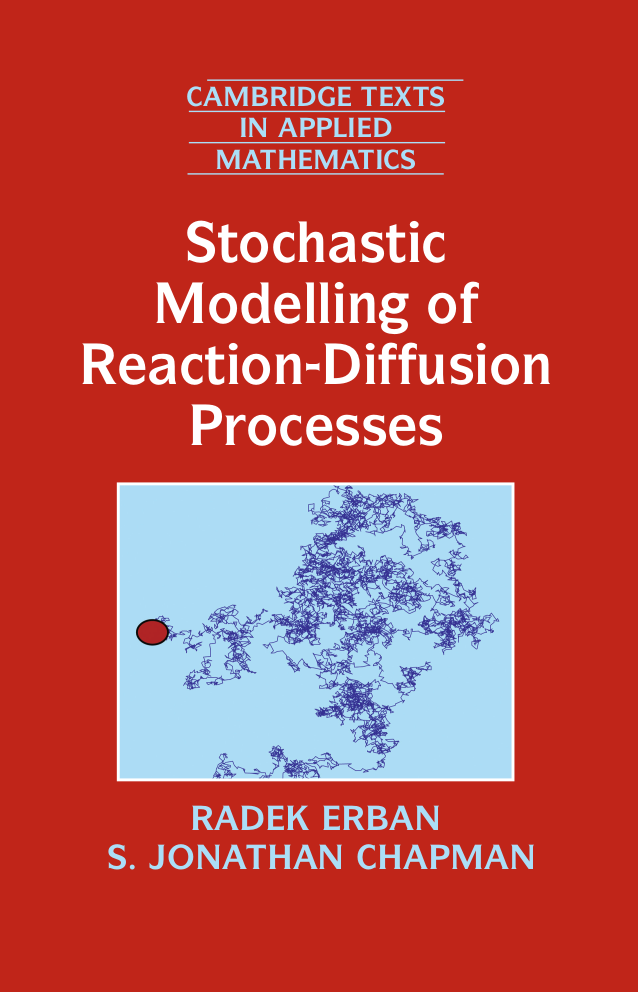Radek Erban and Jonathan Chapman, "Stochastic Modelling of Reaction-Diffusion Processes", Cambridge Texts in Applied Mathematics, Cambridge University Press, 308 pages (2020)Our new book provides an introduction to stochastic methods for modelling biological systems, covering a number of applications, ranging in size from molecular dynamics simulations of small biomolecules to stochastic modelling of groups of animals. The focus is on the underlying mathematics, i.e. it is not assumed that the reader took any advanced courses in biology or chemistry. The book can be used both for self-study and as a supporting text for advanced undergraduate or beginning graduate-level courses in applied mathematics. It discusses the essence of mathematical methods which appear (under different names) in a number of interdisciplinary scientific fields bridging mathematics and computations with biology and chemistry. New mathematical approaches and their analysis are explained using simple examples of biological models. The book starts with stochastic modelling of chemical reactions, introducing stochastic simulation algorithms and mathematical methods for analysis of stochastic models. Different stochastic spatio-temporal models are then studied, including models of diffusion and stochastic reaction-diffusion modelling. The methods covered include molecular dynamics, Brownian dynamics, velocity jump processes and compartment-based models.

Online Course

An online course (with 21 videos) is available on a dedicated YouTube channel. The videos are based on lectures, which were originally prepared for the 2021 course on "Stochastic Modelling of Biological Processes", taken by advanced undergraduate and beginning graduate students of applied mathematics in the Mathematical Institute, University of Oxford. This course covers Chapters 1, 2, 3 and 4 in full detail, and some selected topics in Chapters 6, 7 and 8. The links to each YouTube video are available here:

Lecture 0 (introduction)
Lecture 1 (Sections 1.1 and 1.2)
Lecture 2 (computer demonstration)
Lecture 3 (Sections 1.3, 1.4 and 1.5)
Lecture 4 (Section 2.1)
Lecture 5 (Section 2.2)
Lecture 6 (Section 2.3)
Lecture 7 (Section 2.4)
Lecture 8 (Sections 3.1 and 3.2)
Lecture 9 (Sections 3.3 and 3.4)
Lecture 10 (Sections 3.5 and 3.6)
Lecture 11 (Sections 3.7 and 3.8)
Lecture 12 (Section 4.1)
Lecture 13 (Section 4.2)
Lecture 14 (Sections 4.3 and 4.6)
Lecture 15 (Sections 4.4 and 4.5)
Lecture 16 (Sections 6.1, 6.3 and 6.8)
Lecture 17 (Sections 6.2 and 6.5)
Lecture 18 (Sections 7.1 and 7.2)
Lecture 19 (Sections 7.3 and 7.4)
Lecture 20 (Chapter 8)

Computer codes are available below. Matlab file "FigureX.m" plots the corresponding Figure X in the book.

Chapter 1

Figure 1.1: Figure1_1a.m, Figure1_1b.m (Matlab files)
Figure 1.2: Figure1_2a.m, Figure1_2b.m (Matlab files)
Figure 1.3: Figure1_3a.m, Figure1_3b.m (Matlab files)
Figure 1.4: Figure1_4.m (Matlab file)
Figure 1.5: Figure1_5_a_b.m, Figure1_5_c_d.m (Matlab files)
YouTube videos: Lecture 0 (introduction), Lecture 1 (Sections 1.1 and 1.2), Lecture 2 (computer demonstration), Lecture 3 (Sections 1.3, 1.4 and 1.5)

Chapter 2

Figure 2.1: Figure2_1.m (Matlab file)
Figure 2.2: Figure2_2a.m, Figure2_2b.m (Matlab files)
Figure 2.3: Figure2_3and2_5a.m (Matlab file)
Figure 2.4: Figure2_4and2_5b.m (Matlab file)
Figure 2.5: Figure2_3and2_5a.m, Figure2_4and2_5b.m (Matlab files)
Figure 2.6: Figure2_6and2_9a.m (Matlab file)
Figure 2.7: Figure2_7.m (Matlab file)
Figure 2.8: Figure2_8and2_9b.m (Matlab file)
Figure 2.9: Figure2_6and2_9a.m, Figure2_8and2_9b.m (Matlab files)
Figure 2.10: Figure2_10a.m, Figure2_10b.m (Matlab files)
Figure 2.11: Figure2_11a.m, Figure2_11b.m (Matlab files)
YouTube videos: Lecture 4 (Section 2.1), Lecture 5 (Section 2.2), Lecture 6 (Section 2.3), Lecture 7 (Section 2.4)

Chapter 3

Figure 3.1: Figure3_1a.m, Figure3_1b.m, Figure3_1c.m, Figure3_1d.m (Matlab files)
Figure 3.2: Figure3_2a.m, Figure3_2b.m (Matlab files), Fortran_Figure3_2b.f90 (Fortran file), data_Figure3_2b.dat (data file)
Figure 3.3: Figure3_3.m (Matlab file), Fortran_Figure3_3b.f90 (Fortran file), data_Figure3_3b_1.dat, data_Figure3_3b_2.dat (data files)
Figure 3.4: Figure3_4a.m, Figure3_4b.m (Matlab files), Fortran_Figure3_4b.f90 (Fortran file), data_Figure3_4b_1.dat, data_Figure3_4b_2.dat (data files)
Figure 3.5: Figure3_5.m (Matlab file), Fortran_Figure3_5a.f90, Fortran_Figure3_5b.f90 (Fortran files), data_Figure3_5a.dat, data_Figure3_5b.dat (data files)
YouTube videos: Lecture 8 (Sections 3.1 and 3.2), Lecture 9 (Sections 3.3 and 3.4), Lecture 10 (Sections 3.5 and 3.6), Lecture 11 (Sections 3.7 and 3.8)

Chapter 4

Figure 4.1: Figure4_1.m (Matlab file)
Figure 4.2: Figure4_2a.m, Figure4_2b.m (Matlab files)
Figure 4.3: Figure4_3a.m, Figure4_3b.m (Matlab files)
Figure 4.4: Figure4_4_a_b.m, Figure4_4c.m, Figure4_4d.m (Matlab files)
Figure 4.5: Figure4_5a.m, Figure4_5b.m, Figure4_5d.m (Matlab files)
Figure 4.6: Figure4_6b.m, Figure4_6c.m, Figure4_6d.m (Matlab files)
Figure 4.7: Figure4_7a.m, Figure4_7b.m (Matlab files)
YouTube videos: Lecture 12 (Section 4.1), Lecture 13 (Section 4.2), Lecture 14 (Sections 4.3 and 4.6), Lecture 15 (Sections 4.4 and 4.5)

Chapter 5

Figure 5.1: Figure5_1.m (Matlab file), Fortran_Figure5_1_a_b_c.f90, Fortran_Figure5_1_a_b_c.f90 (Fortran files), data_Figure5_1_a_b_c.dat, data_Figure5_1_a_b_c.dat (data files)
Figure 5.2: Figure5_2.m (Matlab file), Fortran_Figure5_2_a.f90, Fortran_Figure5_2_b.f90, Fortran_Figure5_2_c_d.f90 (Fortran files), data_Figure5_2_a.dat, data_Figure5_2_b.dat, data_Figure5_2_c_d.dat (data files)

Chapter 6

Figure 6.1: Figure6_1.m (Matlab file), Fortran_Figure6_1.f90 (Fortran file), data_Figure6_1.dat (data file)
Figure 6.2: Figure6_2.m (Matlab file), Fortran_Figure6_2.f90 (Fortran file), data_Figure6_2.dat (data file)
Figure 6.3: Figure6_3.m (Matlab file), Fortran_Figure6_3.f90 (Fortran file), data_Figure6_3.dat (data file)
Figure 6.4: Figure6_4.m (Matlab file), Fortran_Figure6_4_a.f90, Fortran_Figure6_4_b.f90 (Fortran files), data_Figure6_4_a.dat, data_Figure6_4_b.dat (data files)
Figure 6.5: Figure6_5.m (Matlab file), Fortran_Figure6_5.f90 (Fortran file), data_Figure6_5.dat (data file)
Figure 6.6: Figure6_4.m (Matlab file), Fortran_Figure6_4_6.f90, Fortran_Figure6_4_6.f90 (Fortran files), data_Figure6_6_a.dat, data_Figure6_6_b.dat (data files)
Figure 6.7: Figure6_7.m (Matlab file), Fortran_Figure6_7_c_d.f90 (Fortran file), data_Figure6_7_c_d.dat (data file)
YouTube videos: Lecture 16 (Sections 6.1, 6.3 and 6.8), Lecture 17 (Sections 6.2 and 6.5)

Chapter 7

Figure 7.1: Figure7_1.m (Matlab file)
Figure 7.2: Figure7_2a.m, Figure7_2b.m (Matlab files), Fortran_Figure7_2_a.f90, Fortran_Figure7_2_b.f90 (Fortran files), data_Figure7_2_a.dat, data_Figure7_2_b.dat (data files)
Figure 7.3: Figure7_3.m (Matlab file), Fortran_Figure7_3.f90 (Fortran file), data_Figure7_3.dat (data file)
Figure 7.4: Figure7_4.m (Matlab file)
Figure 7.5: Figure7_5.m (Matlab file), Fortran_Figure7_5b.f90, Fortran_Figure7_2c.f90 (Fortran files), data_Figure7_5_b.dat, data_Figure7_5_c.dat (data files)
Figure 7.6: Figure7_6.m (Matlab file), Fortran_Figure7_6_a.f90, Fortran_Figure7_6_b.f90 (Fortran files), data_Figure7_6_a.dat, data_Figure7_6_b.dat (data files)
YouTube videos: Lecture 18 (Sections 7.1 and 7.2), Lecture 19 (Sections 7.3 and 7.4)

Chapter 8

Figure 8.1: Figure8_1.m (Matlab file)
Figure 8.2: Figure8_2.m (Matlab file), Fortran_Figure8_2_a.f90, Fortran_Figure8_2_b.f90 (Fortran files), data_Figure8_2_a.dat, data_Figure8_2_b.dat (data files)
Figure 8.3: Figure8_3.m (Matlab file)
Figure 8.5: Figure8_5.m (Matlab file)
Figure 8.6: Figure8_6.m (Matlab file), data_Figure8_6_b.dat, data_Figure8_6_c.dat, data_Figure8_6_d.dat, data_Figure8_6_f.dat (data files)